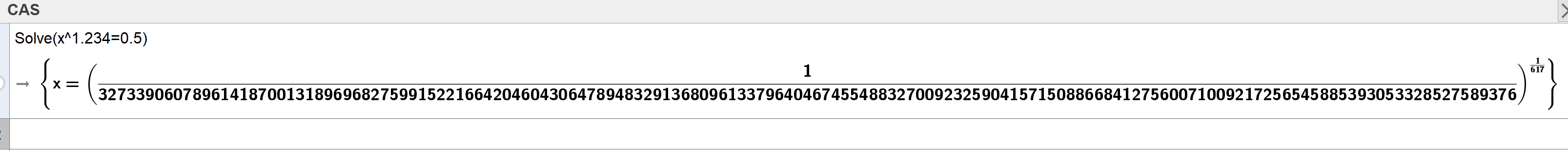# CAS unable to solve simple power equation

alexperl shared this problem 2 years ago
Not a Problem

Trying to solve the equation "x^1.234=0.5" should definitely not be something that a CAS is unable to do.

When typing

`Solve(x^1.234=0.5)`

, I would expect the output to be something like this:

`{x=0.5^(1/1.234)}`

However, that seems to be out of GeoGebra CAS's capabilities:And, if I add another few decimals, like

`Solve(x^1.234567=0.5)`

, then it doesn't even try anymore:PS: Please don't bother telling me that "NSolve" works fine - I know that, and I don't care. The problem is that "Solve" doesn"t work, when it definitely should be.

Or, if that fails, then at the very least internally pass the equation to "NSolve", so that the user at least gets some kind of valuable answer (be it exact or numeric).

However, not giving any answer at all is rather embarrassing.1

One way to cope with this problem would be: "If the algorithm fails to produce a result (either 'Calculation took too long' or '?'), then instead try to replace the numbers by temporary variables (a, b, ...), solve the equation x^a=b numerically for x, and in the end plug in the original numbers a=1.234567 and b=0.5"1

Works fine for me. Do you really need an exact answer to this?1

Replace "1.234" with "1.23456" or "1.234567" and try again.

Apart from that, you're totally missing the point, or otherwise willingly misunderstanding the meaning of "exact". It does not mean "write it in the most complicated and verbose form thinkable", but "output the result without any inherent rounding errors".

In this case that would obviously be

`{x=0.5^(1/1.234)}`

or (considering GeoGebra's notorious dislike of decimal numbers, even if the input contains several - which any other mathematical software correctly interprets as "give me a rounded result!"):

`{x=1/2^(500/617)}"`

As mentioned, everything necessary for thisbehaviour is already there, as can be seen here (again, the "1.234567" fails):So, if floating point numbers are to be avoided at any cost (whatevery your reasons for that might be), why not at least give a reasonable output like the one produced by solving symbolically and then replacing by the given numbers?

Why would anybody want the unreadable output you posted?1

So, in essence: Compare these two and tell me which one you find more user-friendly and suitable for students:

GeoGebra:Mathematica:1

Sorry, we're not able to improve that (due to the design, for better or worse).